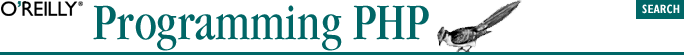home | O'Reilly's CD bookshelfs | FreeBSD | Linux | Cisco | Cisco Exam## 3.6. Variable Functions

```switch(\$which) {
case 'first':
first( );
break;

case 'second':
second( );
break;

case 'third':
third( );
break;
}```

In this case, we could use a variable function call to call the appropriate function. To make a variable function call, include the parameters for a function in parentheses after the variable. To rewrite the previous example:

`\$which();  // if \$which is "first" the function first( ) is called, etc...`

If no function exists for the variable, a runtime error occurs when the code is evaluated. To prevent this, you can use the built-in function function_exists( ) to determine whether a function exists for the value of the variable before calling the function:

`\$yes_or_no = function_exists(function_name);`

For example:

```if(function_exists(\$which)) {
\$which();  // if \$which is "first" the function first( ) is called, etc...
}```

Language constructs such as echo( ) and isset( ) cannot be called through variable functions:

```\$f = 'echo';
\$f('hello, world');  // does not work```